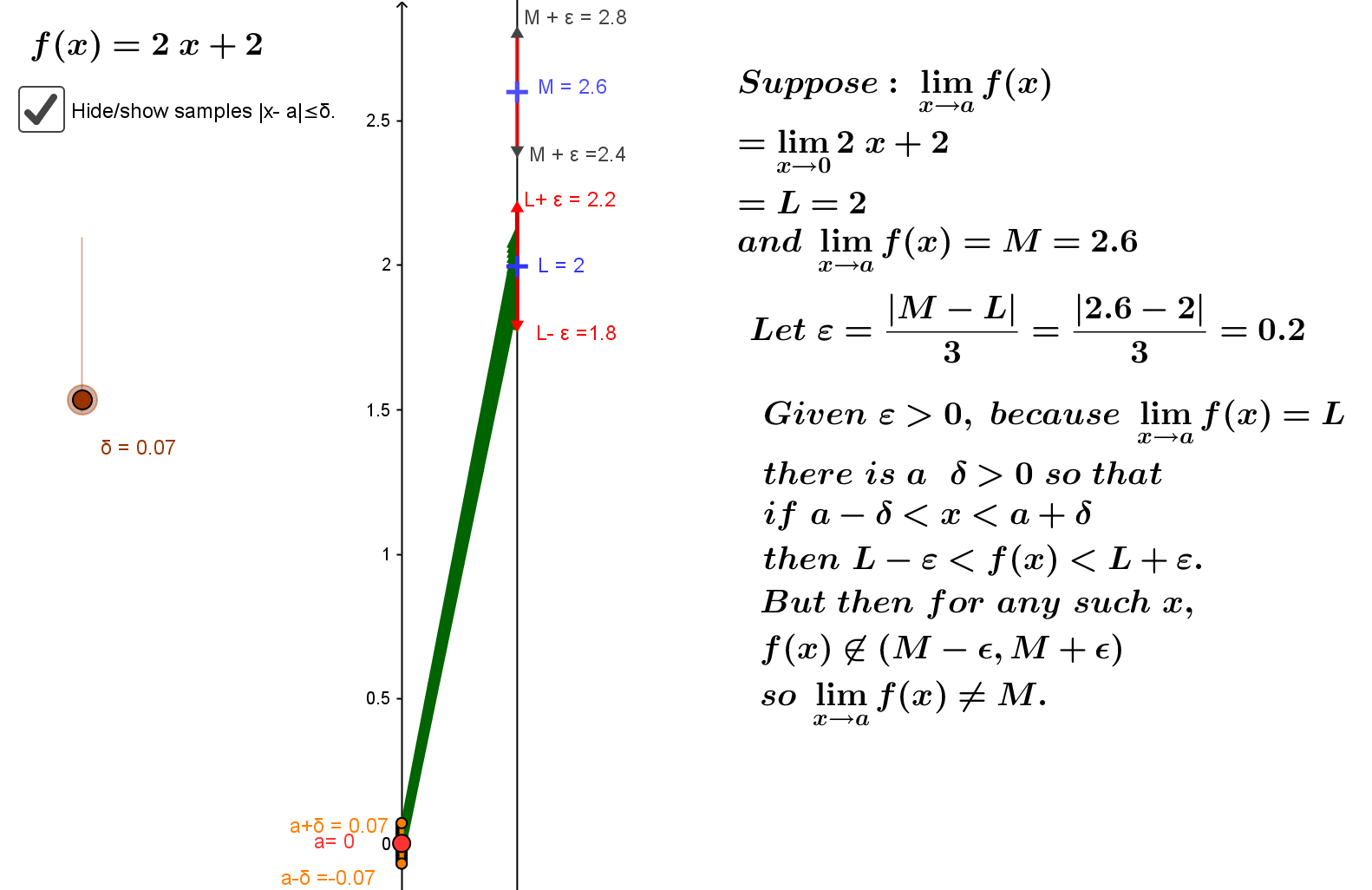Theorem: CCD.UL: If $\lim_{x→a}f(x)=L$ and $\lim_{x→a}f(x)=M$ then $L=M$.Preface to Proof: The argument will be indirect. Assuming that $L \ne M$ it will be demonstrated that $\lim_{x \to a}f(x) \ne M$.

Proof: Assume $L \ne M$. Let $\epsilon = \frac{|L-M|}3$.

Since $\lim_{x→a}f(x)=L$ there is a $\delta > 0$ where is $a-\delta<x<a+\delta, x\ne a$ then $L-\epsilon<f(x)<L+\epsilon$.
But then for any such $x$,  f(x)  is not an element of $(M-\epsilon, M+\epsilon)$ so $\lim_{x \to a}f(x) \ne M$.  EOP

Below is a GeoGebra visualization of this argument using mapping diagrams applied to $f(x)= 2x+2, a =0, L=2$ and $M =2.6.$

You can change the values of $a$, $M$, and $L$, as well as the function $f$ to explore this argument further with other examples.

Martin Flashman, 20 Jan 2018, Created with GeoGebra